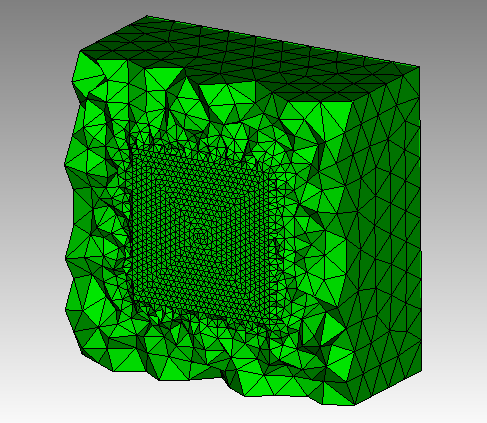# How to create a surface inside a volume and create a conforming mesh to this surface

Hi,

I would like to learn how to include an internal surface within a volume, such that meshing the volume conforms to this internal surface.

Below is a minimum working example. The idea is that I would like that the surface “InnerPlane” becomes part of the volume, such that upon meshing the volume the mesh conforms to this inner surface. Note that I do not want to modify the inner surface geometry.

Could you please let me know how I can achieve this? Would `imprint` and `merge` help? How?

Thank you,

``````cubit.cmd("brick x 10 y 10 z 10")
cubit.cmd("Surface 6  copy scale x 0.0001 y 0.5 z 0.5 ")
surfaceNumber=cubit.get_last_id("surface")
cubit.cmd("surface {} rename 'InnerPlane'".format(surfaceNumber))``````

Hi Josimar,

There are a couple of ways to do this if you want a tetmesh of the body. The easiest way is to mesh it as a non-manifold body. You can do a boolean subtract of the sheet body and the volume to create the non-manifold body. For example,

cubit.cmd(“reset”)
cubit.cmd(“brick x 10 y 10 z 10”)
cubit.cmd("Surface 6 copy scale x 0.0001 y 0.5 z 0.5 ")
surfaceNumber=cubit.get_last_id(“surface”)
cubit.cmd(“surface {} rename ‘InnerPlane’”.format(surfaceNumber))

bodyNumber=cubit.get_last_id(“body”)
cubit.cmd(f’subtract body {bodyNumber} from body 1’)
cubit.cmd(‘compress’)
cubit.cmd(‘surf with name “InnerPlane” size .2’)
cubit.cmd(‘mesh vol 1’)
cubit.cmd(‘vol 1 scheme tetmesh’)
cubit.cmd(‘mesh vol 1’)
cubit.cmd(‘draw tet all’)

Is this what you want?

KarlHi @karl,

Thank you very much for this. This is what I needed.

Before we close this, I wanted to ask you how did you figure out that the subtract command would create one single body with the surface inside?

The reason that I am asking this is because when I read the Cubit manual regarding the `subtract` command (see below) I do not get the impression that subtracting the `InnerPlane` from the volume would result in the `InnerPlane` to be included within the volume. Could you clarify?

Thanks,

Hi,

I will admit that I was a bit surprised when one of the Sandia developers shared this with me. I was even a little more surprised when they told me that the tet-mesher now handles non-manifold volumes correctly.

It looks like the documentation should be updated to reflect how non-manifold bodies are handled.

Thanks for pointing that out.
Karl

Thank you @karl for clarifying!

Best,

Hi @karl,

A quick follow up question: I am noticing that when I have several internal surfaces and with multivolumes, the subtract command changes the volume name and id of the original master volume.

In practice it means that in the command below, the id associated with body 1 is changing. I know this is a long shot, but would you know a way to prevent that the body 1 id changes?

Thanks,

`cubit.cmd(f’subtract body {bodyNumber} from body 1’)`

Hi,

I saw that it was doing that. Hence, the compress in my original example. I did notice that if you name volume 1 at the body level instead of the volume level, the name sticks.

body 1 name “my_body”

Typically, body ids and volume ids are the same. You may want to use the parse_cubit_list method to make sure you have the right body id.

body_id = cubit.parse_cubit_list(“body”, “in volume 1”)
cubit.cmd( f"body {body_id} name “my_body”)

You could then do the inverse to get the volume out. You need the right volume id to mesh.

volume_id = cubit.parse_cubit_list(“volume” "in body with name ‘my_body’ ")

Does that help?

Karl